# 量子霍尔效应

## 一、什么是霍尔效应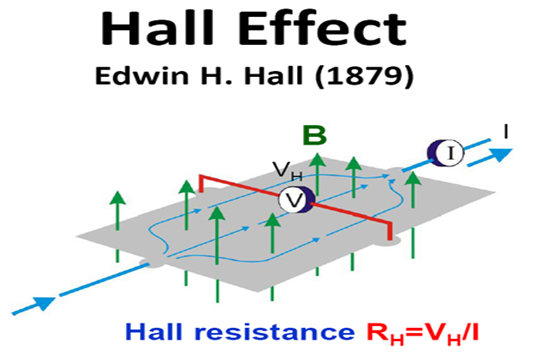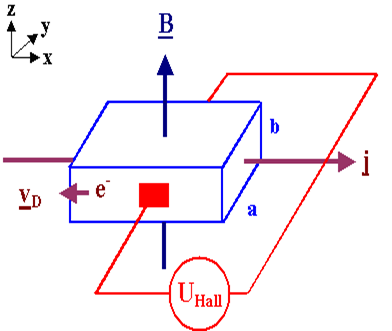$$U_{Hall}\varpropto B*I$$

$$U_{Hall}=E*a=Bv*a=B\frac{I}{nqab} a=\frac{B}{nqb}I=\frac{B}{n_sq} I$$
$R_H\equiv \frac{ B}{n_sq}$定义为霍尔电阻，这里的$n_s$是载流子的面密度，它在实验上可以很容易调整，电流I一般用恒流源控制，不轻易改变。

## 二、量子霍尔效应的实验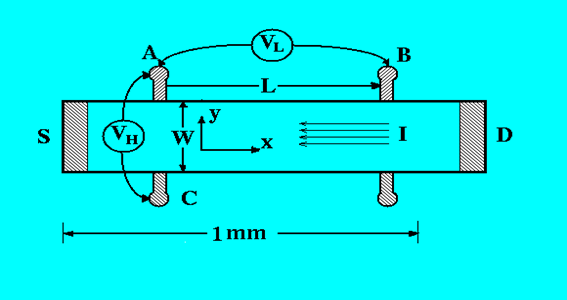### 1、二维平面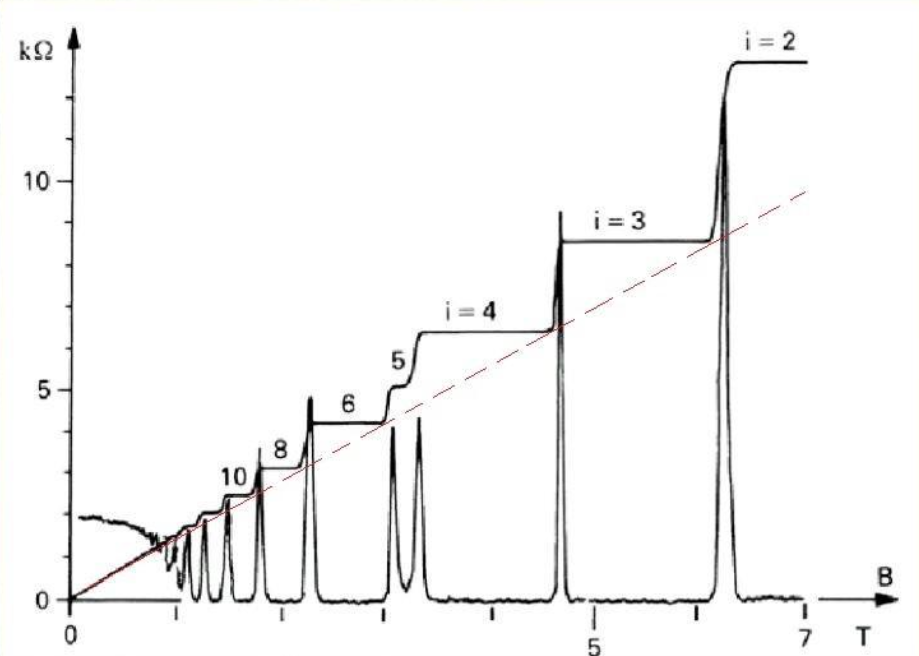### 2、如何控制电流、电子面密度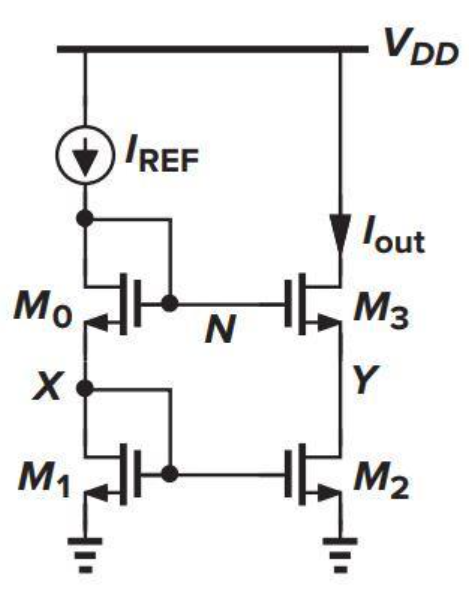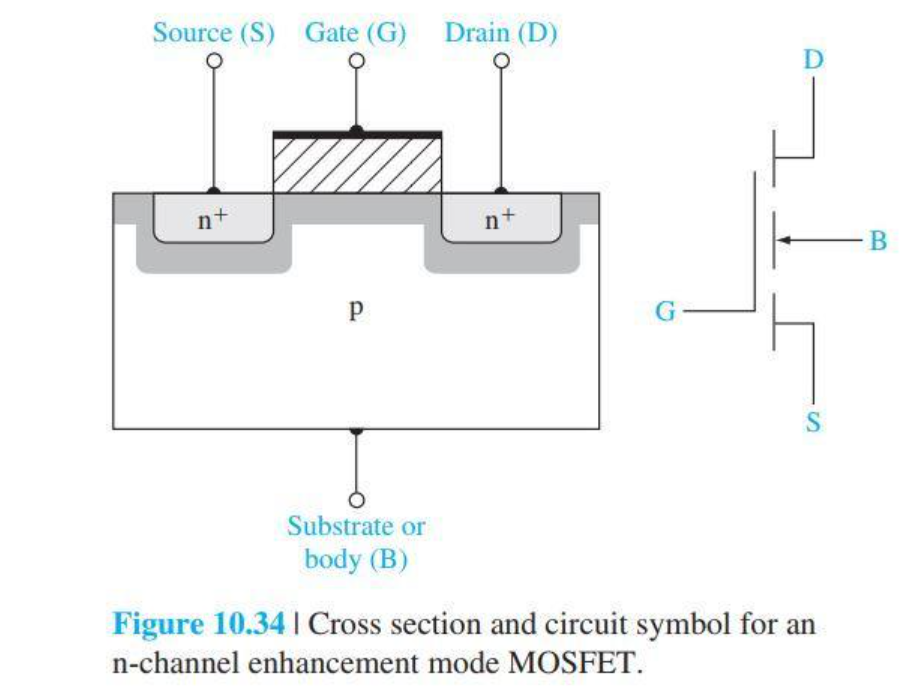$$n_s=C_{ox}*(V_{gs}-V_t)$$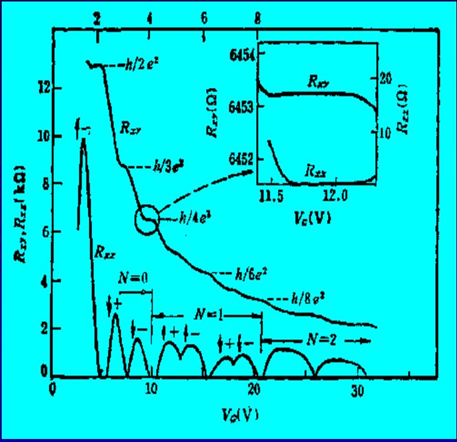• 为什么霍尔电阻$R_{xy}$会出现平台？
• 为什么水平电阻$R_{xx}$会有出现零的状态？
• 电子在平台期间呈现什么奇特的运动状态？

## 三、直观物理图像

### 1、磁场下的圆周运动

$$m\frac{v^2}{r} = qvB\Rightarrow r=\frac{mv}{Bq}$$

$$2\pi r=N\lambda=N\frac{h}{mv}\Rightarrow mv^2=N\hbar \omega,N=1,2,3...$$
$\omega \equiv \frac{Bq}{m},\hbar \equiv \frac{h}{2\pi}$可以看出，量子化后的电子呈现分立能级，那么每一个能级能容纳多少电子呢？这就是能级的简并度，类似一层楼能容纳多少人。

### 2、二维平面的能量密度

$$\vec{ \kappa} = \frac{2 \pi}{W}\vec{i}+\frac{2 \pi}{L}\vec{j}$$

$$E= \frac{ \hbar^2 \kappa^2}{2m}$$

$$G(E)dE=G(\kappa)d\kappa=\frac{2\pi \kappa d\kappa}{2 \pi/W *2 \pi/L}$$

$$g(E)=\frac{G(E)}{WL}=\frac{2\pi \kappa }{2 \pi *2 \pi} \frac{1}{dE/d\kappa}=\frac{m}{2 \pi \hbar^2}$$

### 3、霍尔电阻量子化

$$g(E)*\hbar \omega=\frac{m}{2 \pi \hbar^2} *\hbar \frac{Bq}{m}=\frac{Bq}{h}$$

$$R_H\equiv \frac{B}{n_sq} =\frac{h}{i*q^2}=\frac{25.8 k\Omega}{i}$$

### 4、详细的过程分析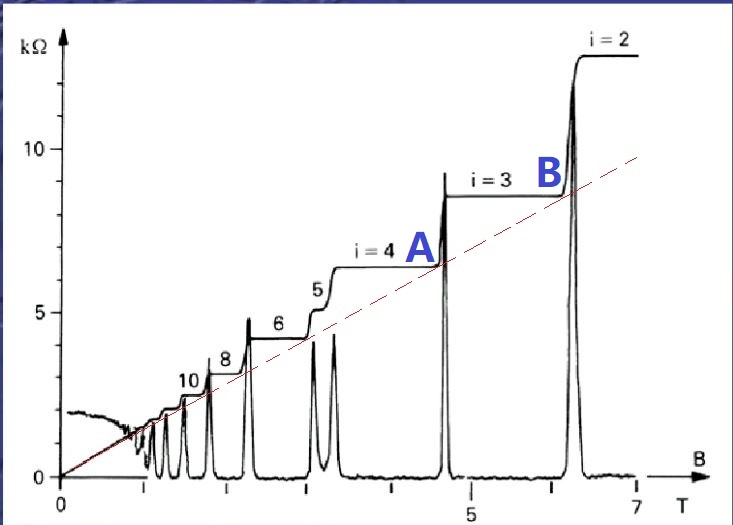## 五、其他

1、霍尔电阻平台的平整度极高，误差为$10^{-8}$，这一特性被用来制定电阻标准。
2、整数霍尔效应之后两年，崔琦等科学家又发现了分数量子霍尔效应，并提出了新的物理图像来解释这一现象。
3、2018年复旦大学的修发贤组在砷化镉材料中发现三维空间的量子霍尔效应。
4、2006年，斯坦福大学张首晟团队提出了量子自旋霍尔效应的理论，意在利用电子自旋和电力的规律，降低能耗，研制新型计算机，并在2009年预言了三种拓扑绝缘体。
5、2013年，清华薛琪坤团队在实验上观测到量子反常霍尔效应，它不同于之前的量子霍尔效应，它不依赖于强磁场而是由材料本身的自发磁化产生，在零磁场下就能实现，不过仍需要低温环境。